# Sparse Gaussian Process Regression¶

Let’s set some setting for this Jupyter Notebook.

In :

%matplotlib inline
from warnings import filterwarnings
filterwarnings("ignore")
import os
os.environ['THEANO_FLAGS'] = 'device=cpu'

import numpy as np
import pandas as pd
import pymc3 as pm
import seaborn as sns
import matplotlib.pyplot as plt
np.random.seed(12345)
rc = {'xtick.labelsize': 20, 'ytick.labelsize': 20, 'axes.labelsize': 20, 'font.size': 20,
'legend.fontsize': 12.0, 'axes.titlesize': 10, "figure.figsize": [12, 6]}
sns.set(rc = rc)
from IPython.core.interactiveshell import InteractiveShell
InteractiveShell.ast_node_interactivity = "all"


Now, let’s import the SparseGaussianProcessRegression algorithm from the pymc-learn package.

In :

import pmlearn
from pmlearn.gaussian_process import SparseGaussianProcessRegressor
print('Running on pymc-learn v{}'.format(pmlearn.__version__))

Running on pymc-learn v0.0.1.rc0


## Step 1: Prepare the data¶

Generate synthetic data.

In :

n = 150 # The number of data points
X = np.linspace(start = 0, stop = 10, num = n)[:, None] # The inputs to the GP, they must be arranged as a column vector

# Define the true covariance function and its parameters
length_scale_true = 1.0
signal_variance_true = 3.0
cov_func = signal_variance_true**2 * pm.gp.cov.ExpQuad(1, length_scale_true)

# A mean function that is zero everywhere
mean_func = pm.gp.mean.Constant(10)

# The latent function values are one sample from a multivariate normal
# Note that we have to call eval() because PyMC3 built on top of Theano
f_true = np.random.multivariate_normal(mean_func(X).eval(),
cov_func(X).eval() + 1e-8*np.eye(n), 1).flatten()

# The observed data is the latent function plus a small amount of Gaussian distributed noise
# The standard deviation of the noise is sigma
noise_variance_true = 2.0
y = f_true + noise_variance_true * np.random.randn(n)

## Plot the data and the unobserved latent function
fig = plt.figure(figsize=(12,5))
ax = fig.gca()
ax.plot(X, f_true, "dodgerblue", lw=3, label="True f");
ax.plot(X, y, 'ok', ms=3, label="Data");
ax.set_xlabel("X"); ax.set_ylabel("y"); plt.legend();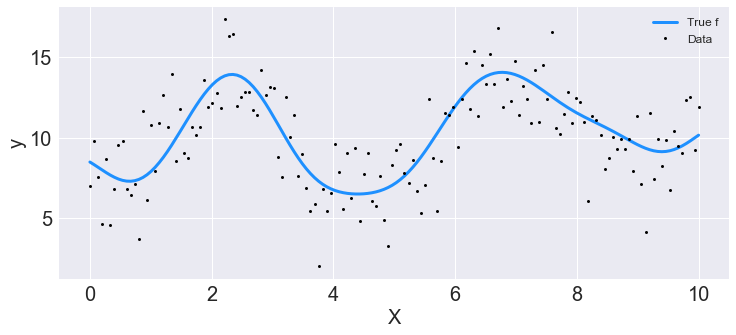In :

from sklearn.model_selection import train_test_split
X_train, X_test, y_train, y_test = train_test_split(X, y, test_size=0.3)


## Step 2: Instantiate a model¶

In :

model = SparseGaussianProcessRegressor()


## Step 3: Perform Inference¶

In :

model.fit(X_train, y_train)

Average Loss = -4,669.3: 100%|██████████| 200000/200000 [06:12<00:00, 536.41it/s]
Finished [100%]: Average Loss = -4,669.6

Out:

SparseGaussianProcessRegressor(prior_mean=0.0)


## Step 4: Diagnose convergence¶

In :

model.plot_elbo()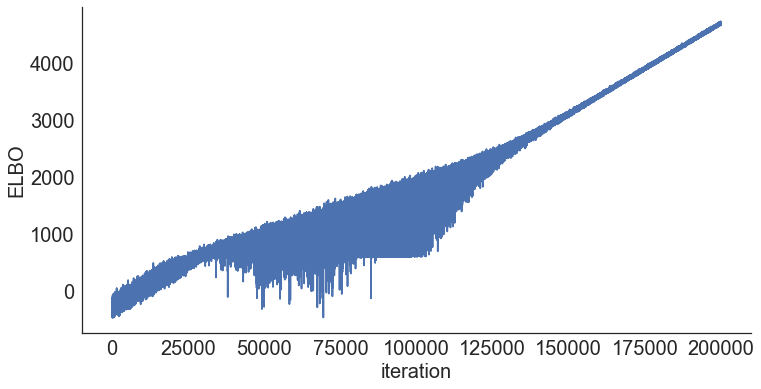In :

pm.traceplot(model.trace);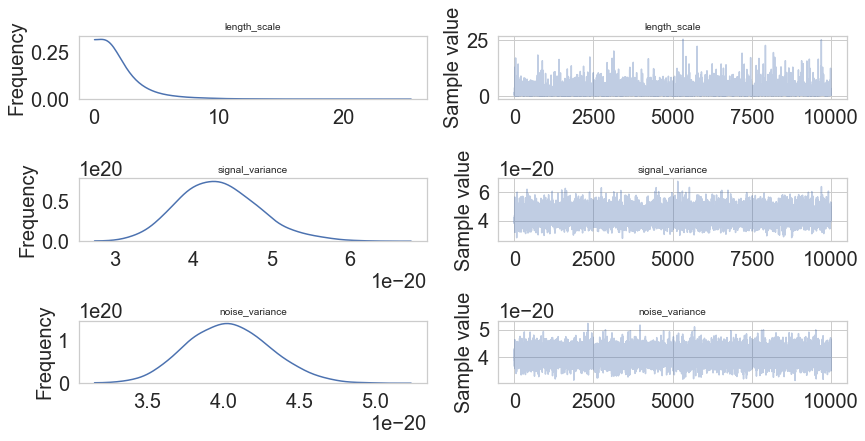In :

pm.traceplot(model.trace, lines = {"signal_variance": signal_variance_true,
"noise_variance": noise_variance_true,
"length_scale": length_scale_true},
varnames=["signal_variance", "noise_variance", "length_scale"]);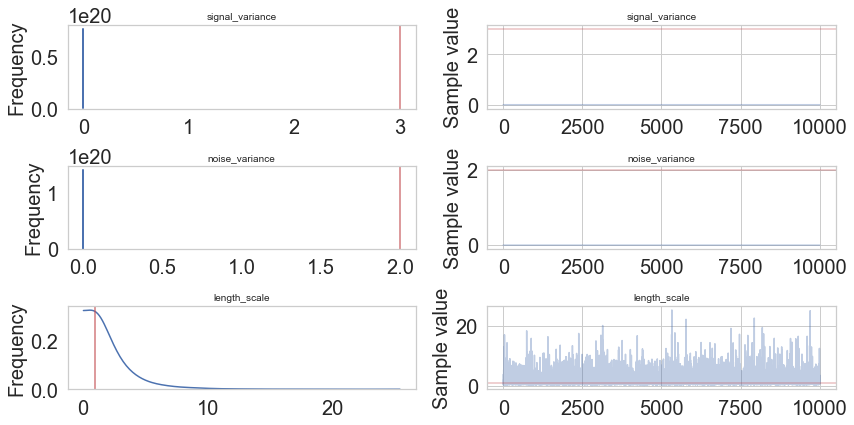In :

pm.forestplot(model.trace, varnames=["signal_variance", "noise_variance", "length_scale"]);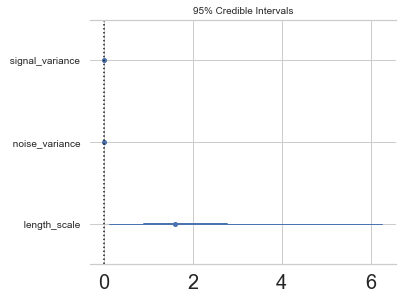## Step 5: Critize the model¶

In :

pm.summary(model.trace, varnames=["signal_variance", "length_scale", "noise_variance"])

Out:

mean sd mc_error hpd_2.5 hpd_97.5
signal_variance__0 2.266128e-20 3.632205e-21 3.731201e-23 1.578536e-20 2.999736e-20
length_scale__0_0 2.233311e+00 2.182089e+00 2.042519e-02 1.187429e-01 6.257235e+00
noise_variance__0 2.216509e-20 3.491209e-21 3.041214e-23 1.571128e-20 2.914439e-20
In :

pm.plot_posterior(model.trace, varnames=["signal_variance", "noise_variance", "length_scale"],
figsize = [14, 8]);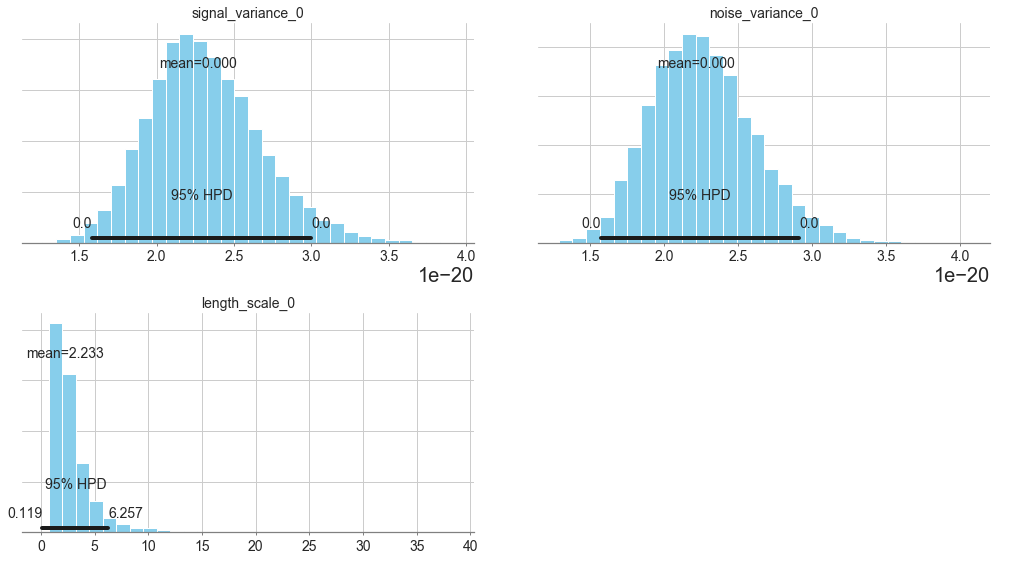In :

# collect the results into a pandas dataframe to display
# "mp" stands for marginal posterior
pd.DataFrame({"Parameter": ["length_scale", "signal_variance", "noise_variance"],
"Predicted Mean Value": [float(model.trace["length_scale"].mean(axis=0)),
float(model.trace["signal_variance"].mean(axis=0)),
float(model.trace["noise_variance"].mean(axis=0))],
"True value": [length_scale_true, signal_variance_true, noise_variance_true]})

Out:

Parameter Predicted Mean Value True value
0 length_scale 2.182521 1.0
1 signal_variance 9.261435 3.0
2 noise_variance 0.002241 2.0

## Step 6: Use the model for prediction¶

In [ ]:

y_predict1 = model.predict(X_test)

In [ ]:

y_predict1

In [ ]:

model.score(X_test, y_test)

In [ ]:

model.save('pickle_jar/sgpr')


### Use already trained model for prediction¶

In [ ]:

model_new = SparseGaussianProcessRegressor()
model_new.score(X_test, y_test)


## Multiple Features¶

In [ ]:

num_pred = 2
X = np.random.randn(1000, num_pred)
noise = 2 * np.random.randn(1000,)
Y = X.dot(np.array([4, 5])) + 3 + noise

In [ ]:

y = np.squeeze(Y)

In [ ]:

model_big = SparseGaussianProcessRegressor()

In [ ]:

model_big.fit(X, y, inference_args={"n" : 1000})

In [ ]:

pm.summary(model_big.trace, varnames=["signal_variance", "length_scale", "noise_variance"])


## MCMC¶

In :

model2 = SparseGaussianProcessRegressor()
model2.fit(X_train, y_train, inference_type='nuts')

Multiprocess sampling (4 chains in 4 jobs)
NUTS: [f_rotated_, noise_variance_log__, signal_variance_log__, length_scale_log__]
100%|██████████| 1500/1500 [00:24<00:00, 60.20it/s]
There were 92 divergences after tuning. Increase target_accept or reparameterize.
There were 88 divergences after tuning. Increase target_accept or reparameterize.
There were 87 divergences after tuning. Increase target_accept or reparameterize.
There were 40 divergences after tuning. Increase target_accept or reparameterize.
The number of effective samples is smaller than 10% for some parameters.
Multiprocess sampling (4 chains in 4 jobs)
NUTS: [f_rotated_, noise_variance_log__, signal_variance_log__, length_scale_log__]
100%|██████████| 4000/4000 [11:41<00:00,  5.70it/s]
There were 4 divergences after tuning. Increase target_accept or reparameterize.
There were 2 divergences after tuning. Increase target_accept or reparameterize.
There were 1 divergences after tuning. Increase target_accept or reparameterize.
There were 1 divergences after tuning. Increase target_accept or reparameterize.

Out:

GaussianProcessRegression()

In :

pm.traceplot(model2.trace, lines = {"signal_variance": signal_variance_true,
"noise_variance": noise_variance_true,
"length_scale": length_scale_true},
varnames=["signal_variance", "noise_variance", "length_scale"]);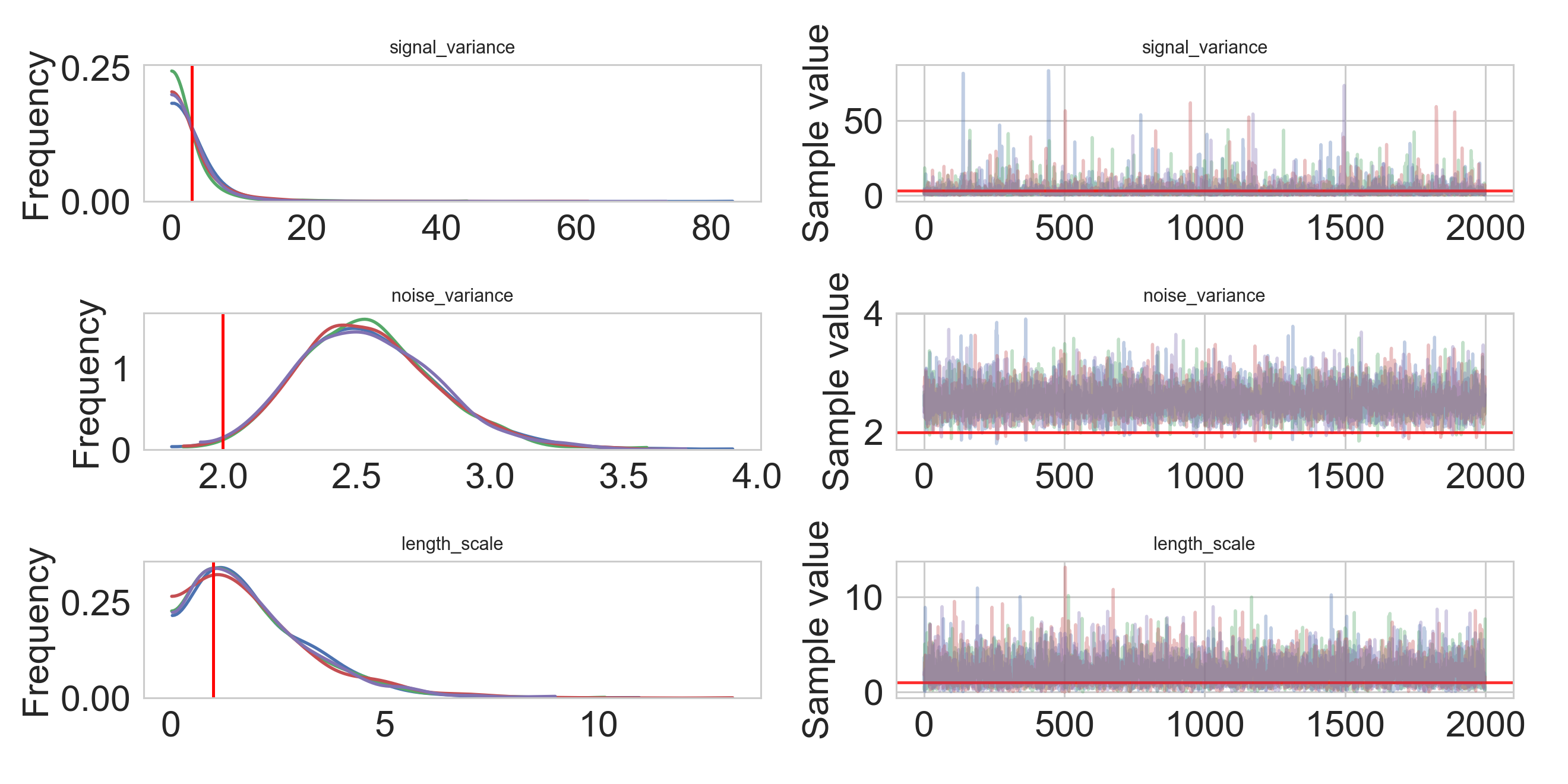In :

pm.gelman_rubin(model2.trace, varnames=["signal_variance", "noise_variance", "length_scale"])

Out:

{'signal_variance': array([ 1.00134827]),
'noise_variance': array([ 0.99982997]),
'length_scale': array([[ 0.9997668]])}

In :

pm.energyplot(model2.trace);